# Tag: monstrous moonshine

In this series I’ll mention some books I found entertaining, stimulating or comforting during these Corona times. Read them at your own risk.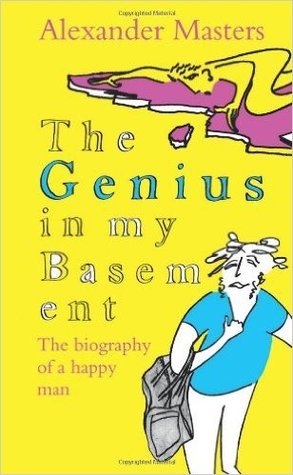This must have been the third time I’ve read The genius in by basement – The biography of a happy man by Alexander masters.

I first read it when it came out in 2011.

Then, in conjunction with Genius at play – The Curious Mind of John Horton Conway Conway’s biography by Siobhan Roberts, in july 2017, which is probably the best way to read this book.

And, then again last week, as Simon Norton‘s work pops up wherever I look, as in the previous post.

It takes some time to get used to the rather chaotic style (probably used because that’s how Masters perceives Norton), and all attempts at explaining Simon’s mathematics can better be skipped.

The book tries to find an answer as to why a child prodigy and genius like Simon Norton failed to secure a safe place in academics.

Page 328:

Simon’s second explanation of his loss of mathematical direction is heartbreaking. Now that Conway has fled to America, there is no one in the mathematical world who will work with him.

They say he is too peculiar, too shabby, too old.

His interests are fixed in mathematics that has had its day. His brilliance is frigid. His talent, perfectly suited to an extraordinary moment in algebraic history (the symmetry work at Cambridge during the early 1970s and 1980s) is out of fashion.

This may give the impression that Norton stopped doing good math after Conway left for Princeton in 1985. This is far from true.

Norton’s Wikipedia page mentions only post 1995 publications, which in itself is deplorable, as it leaves out his contributions to the ATLAS and his seminal paper with Conway on Monstrous moonshine.

Here’s Alexander Masters talking about ‘Genius in my basement’

I’ll leave you with a nice quote, comparing Monstrous Moonshine to a Sainsbury’s bag on Jupiter.

Page 334:

This much I do know: Monstrous Moonshine links the Monster to distant mathematics and the structure of space in ways that are as awe-inspiring to a man like Simon as it would be to an astronaut to step out of his space machine on Jupiter, and find a Sainsbury’s bag floating past. That’s why it’s called ‘Moonshine’, because mathematicians can even now hardly believe it.

‘I think’, said Simon, standing up from his berth and shaking crumbs and clotted blobs of oil and fish off his T-shirt onto the covers, ‘I can explain to you what Moonshine is in one sentence.’

When he really tries, Simon can be a model of clarity.

‘It is,’ he said, ‘the voice of God.’

Ps, wrt. SNORT.

Let’s try to identify the $\Psi(n) = n \prod_{p|n}(1+\frac{1}{p})$ points of $\mathbb{P}^1(\mathbb{Z}/n \mathbb{Z})$ with the lattices $L_{M \frac{g}{h}}$ at hyperdistance $n$ from the standard lattice $L_1$ in Conway’s big picture.

Here are all $24=\Psi(12)$ lattices at hyperdistance $12$ from $L_1$ (the boundary lattices):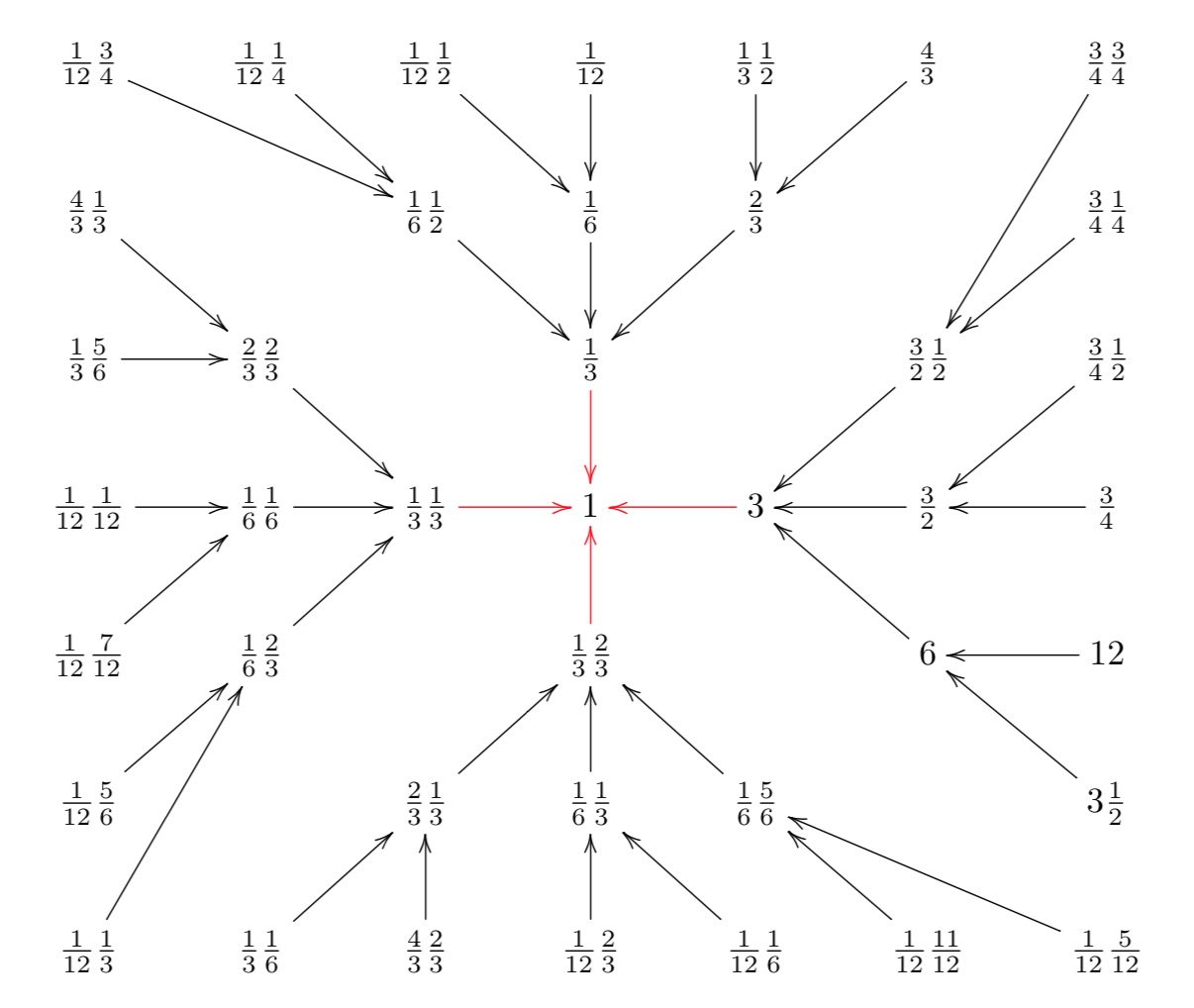You can also see the $4 = \Psi(3)$ lattices at hyperdistance $3$ (those connected to $1$ with a red arrow) as well as the intermediate $12 = \Psi(6)$ lattices at hyperdistance $6$.

The vertices of Conway’s Big Picture are the projective classes of integral sublattices of the standard lattice $\mathbb{Z}^2=\mathbb{Z} e_1 \oplus \mathbb{Z} e_2$.

Let’s say our sublattice is generated by the integral vectors $v=(v_1,v_2)$ and $w=(w_1.w_2)$. How do we determine its class $L_{M,\frac{g}{h}}$ where $M \in \mathbb{Q}_+$ is a strictly positive rational number and $0 \leq \frac{g}{h} < 1$?

Here’s an example: the sublattice (the thick dots) is spanned by the vectors $v=(2,1)$ and $w=(1,4)$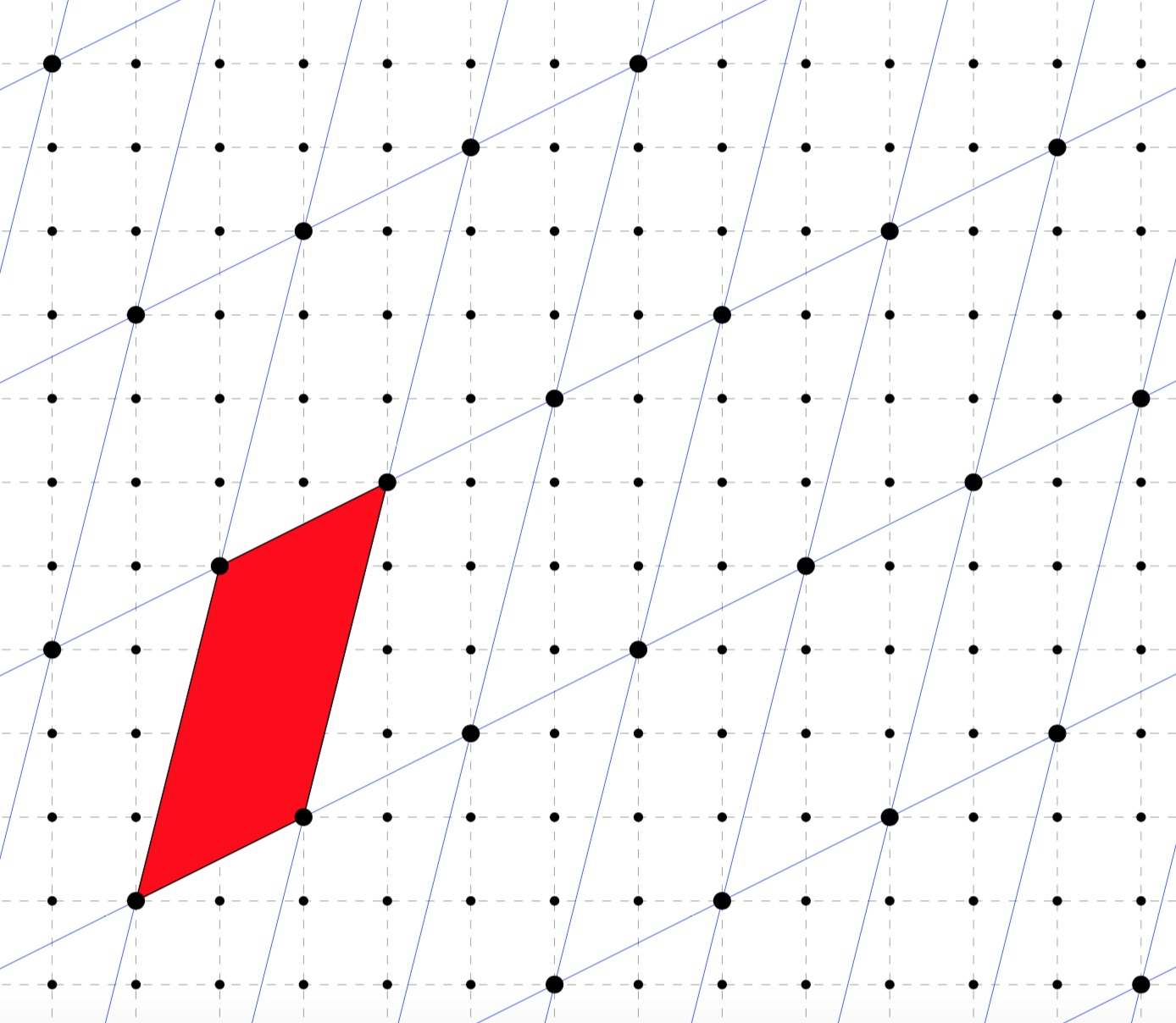Well, we try to find a basechange matrix in $SL_2(\mathbb{Z})$ such that the new 2nd base vector is of the form $(0,z)$. To do this take coprime $(c,d) \in \mathbb{Z}^2$ such that $cv_1+dw_1=0$ and complete with $(a,b)$ satisfying $ad-bc=1$ via Bezout to a matrix in $SL_2(\mathbb{Z})$ such that
$\begin{bmatrix} a & b \\ c & d \end{bmatrix} \begin{bmatrix} v_1 & v_2 \\ w_1 & w_2 \end{bmatrix} = \begin{bmatrix} x & y \\ 0 & z \end{bmatrix}$
then the sublattice is of class $L_{\frac{x}{z},\frac{y}{z}~mod~1}$.

In the example, we have
$\begin{bmatrix} 0 & 1 \\ -1 & 2 \end{bmatrix} \begin{bmatrix} 2 & 1 \\ 1 & 4 \end{bmatrix} = \begin{bmatrix} 1 & 4 \\ 0 & 7 \end{bmatrix}$
so this sublattice is of class $L_{\frac{1}{7},\frac{4}{7}}$.

Starting from a class $L_{M,\frac{g}{h}}$ it is easy to work out its hyperdistance from $L_1$: let $d$ be the smallest natural number making the corresponding matrix integral
$d. \begin{bmatrix} M & \frac{g}{h} \\ 0 & 1 \end{bmatrix} = \begin{bmatrix} u & v \\ 0 & w \end{bmatrix} \in M_2(\mathbb{Z})$
then $L_{M,\frac{g}{h}}$ is at hyperdistance $u . w$ from $L_1$.

Now that we know how to find the lattice class of any sublattice of $\mathbb{Z}^2$, let us assign a class to any point $[c:d]$ of $\mathbb{P}^1(\mathbb{Z}/n\mathbb{Z})$.

As $gcd(c,d)=1$, by Bezout we can find a integral matrix with determinant $1$
$S_{[c:d]} = \begin{bmatrix} a & b \\ c & d \end{bmatrix}$
But then the matrix
$\begin{bmatrix} a.n & b.n \\ c & d \end{bmatrix}$
has determinant $n$.

Working backwards we see that the class $L_{[c:d]}$ of the sublattice of $\mathbb{Z}^2$ spanned by the vectors $(a.n,b.n)$ and $(c,d)$ is of hyperdistance $n$ from $L_1$.

This is how the correspondence between points of $\mathbb{P}^1(\mathbb{Z}/n\mathbb{Z})$ and classes in Conway’s big picture at hyperdistance $n$ from $L_1$ works.

Let’s do an example. Take the point $[7:3] \in \mathbb{P}^1(\mathbb{Z}/12\mathbb{Z})$ (see last time), then
$\begin{bmatrix} -2 & -1 \\ 7 & 3 \end{bmatrix} \in SL_2(\mathbb{Z})$
so we have to determine the class of the sublattice spanned by $(-24,-12)$ and $(7,3)$. As before we have to compute
$\begin{bmatrix} -2 & -7 \\ 7 & 24 \end{bmatrix} \begin{bmatrix} -24 & -12 \\ 7 & 3 \end{bmatrix} = \begin{bmatrix} -1 & 3 \\ 0 & -12 \end{bmatrix}$
giving us that the class $L_{[7:3]} = L_{\frac{1}{12}\frac{3}{4}}$ (remember that the second term must be taken $mod~1$).

If you do this for all points in $\mathbb{P}^1(\mathbb{Z}/12\mathbb{Z})$ (and $\mathbb{P}^1(\mathbb{Z}/6\mathbb{Z})$ and $\mathbb{P}^1(\mathbb{Z}/3 \mathbb{Z})$) you get this version of the picture we started with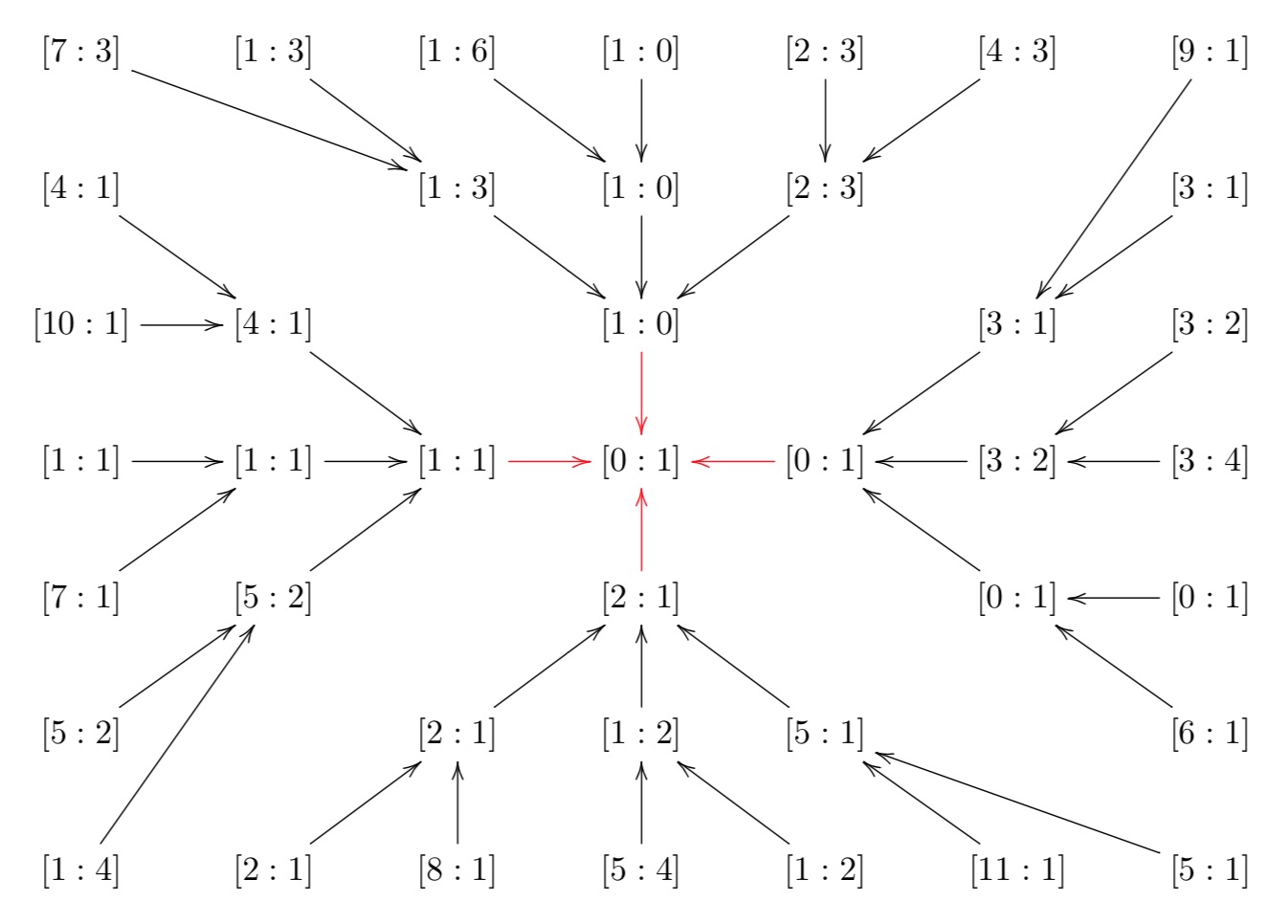You’ll spot that the preimages of a canonical coordinate of $\mathbb{P}^1(\mathbb{Z}/m\mathbb{Z})$ for $m | n$ are the very same coordinate together with ‘new’ canonical coordinates in $\mathbb{P}^1(\mathbb{Z}/n\mathbb{Z})$.

To see that this correspondence is one-to-one and that the index of the congruence subgroup
$\Gamma_0(n) = \{ \begin{bmatrix} p & q \\ r & s \end{bmatrix}~|~n|r~\text{and}~ps-qr=1 \}$
in the full modular group $\Gamma = PSL_2(\mathbb{Z})$ is equal to $\Psi(n)$ it is useful to consider the action of $PGL_2(\mathbb{Q})^+$ on the right on the classes of lattices.

The stabilizer of $L_1$ is the full modular group $\Gamma$ and the stabilizer of any class is a suitable conjugate of $\Gamma$. For example, for the class $L_n$ (that is, of the sublattice spanned by $(n,0)$ and $(0,1)$, which is of hyperdistance $n$ from $L_1$) this stabilizer is
$Stab(L_n) = \{ \begin{bmatrix} a & \frac{b}{n} \\ c.n & d \end{bmatrix}~|~ad-bc = 1 \}$
and a very useful observation is that
$Stab(L_1) \cap Stab(L_n) = \Gamma_0(n)$
This is the way Conway likes us to think about the congruence subgroup $\Gamma_0(n)$: it is the joint stabilizer of the classes $L_1$ and $L_n$ (as well as all classes in the ‘thread’ $L_m$ with $m | n$).

On the other hand, $\Gamma$ acts by rotations on the big picture: it only fixes $L_1$ and maps a class to another one of the same hyperdistance from $L_1$.The index of $\Gamma_0(n)$ in $\Gamma$ is then the number of classes at hyperdistance $n$.

To see that this number is $\Psi(n)$, first check that the classes at hyperdistance $p^k$ for $p$ a prime number and for all $k$ for the $p+1$ free valent tree with root $L_1$, so there are exactly $p^{k-1}(p+1)$ classes as hyperdistance $p^k$.

To get from this that the number of hyperdistance $n$ classes is indeed $\Psi(n) = \prod_{p|n}p^{v_p(n)-1}(p+1)$ we have to use the prime- factorisation of the hyperdistance (see this post).

The fundamental domain for the action of $\Gamma_0(12)$ by Moebius tranfos on the upper half plane must then consist of $48=2 \Psi(12)$ black or white hyperbolic triangles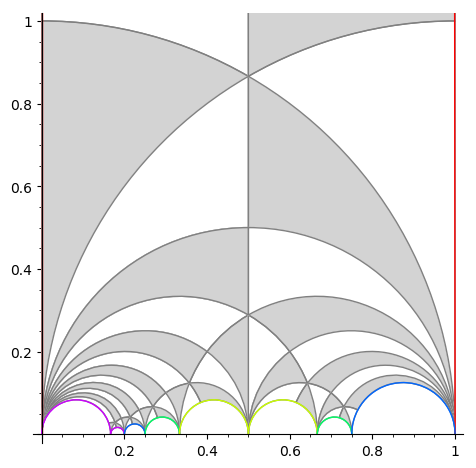Next time we’ll see how to deduce the ‘monstrous’ Grothendieck dessin d’enfant for $\Gamma_0(12)$ from it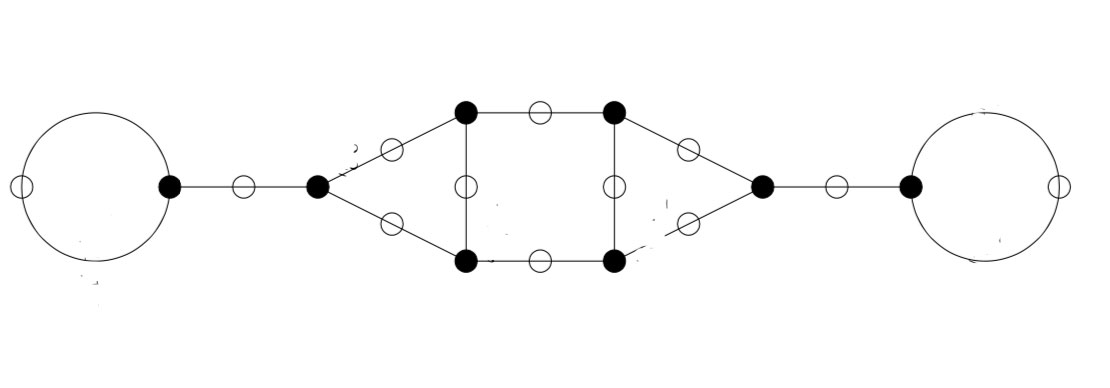We’re slowly closing in on the elusive moonshine picture, which is the subgraph of Conway’s Big Picture needed to describe all 171 moonshine groups.

About nine years ago I had a first go at it, drawing a tiny fraction of it, just enough to understand the 9 moonshine groups appearing in Duncan’s realization of McKay’s E(8)-observation.

Over the last weeks I’ve made enough doodles to feel confident that the full picture is within reach and is less unwieldy than I once feared it might be.

The moonshine picture only involves about 212 lattices and there are about 97 snakes crawling into it, the dimension of the largest cell being 3.

I write ‘about’ on purpose as I may have forgotten a few, or counted some twice as is likely to happen in all projects involving a few hundreds of things. I’ll come back to it later.

For now, I can only show you the monstrous moonshine painting, which is a work by the Chilean artist Magdalena Atria.

Here’s a close up: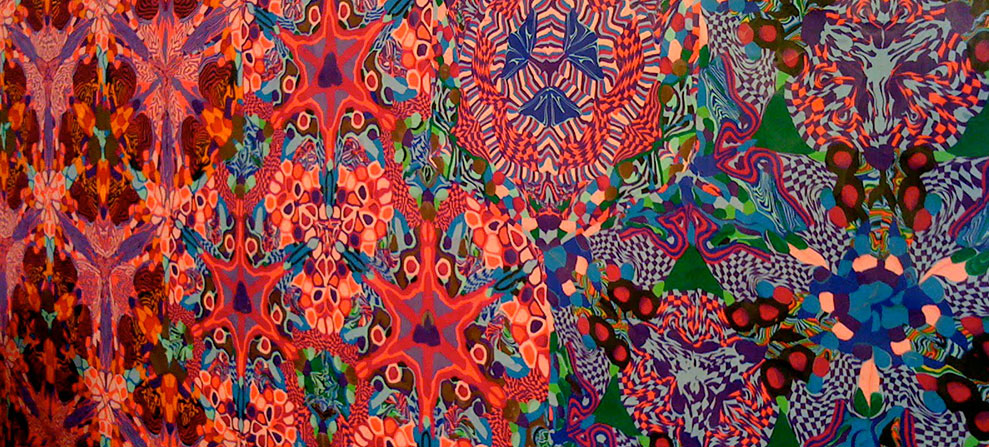It is a large scale painting made with plasticine, directly attached to the wall of the Alejandra Von Hartz gallery where it was exhibited in 2010.What does it have to do with monstrous moonshine?

From the press release:

“In mathematics ‘monstrous moonshine’ is a term devised by John H. Conway and Simon P. Norton in 1979, used to describe the (then totally unexpected) connection between the monster group M and modular functions.

The term ‘monstrous moonshine’ was picked to convey the feelings from the bizarre relations between seemingly unrelated structures. The same spirit of connecting apparently unrelated situations, at times revealing deeper links and at times constructing them, permeates through Atria’s work in this exhibition.”

I was impressed by the first sentence until I read the Wikipedia article on monstrous moonshine which starts off with:

“In mathematics, monstrous moonshine, or moonshine theory, is the unexpected connection between the monster group M and modular functions, in particular, the j function. The term was coined by John Conway and Simon P. Norton in 1979.”

It appears that curators of art-exhibitions, and the intended public of their writings, are familiar with modular forms and functions, but fail to grasp the $j$-function.

When they speak about ‘modular forms’, I fear they’re thinking of something entirely different.